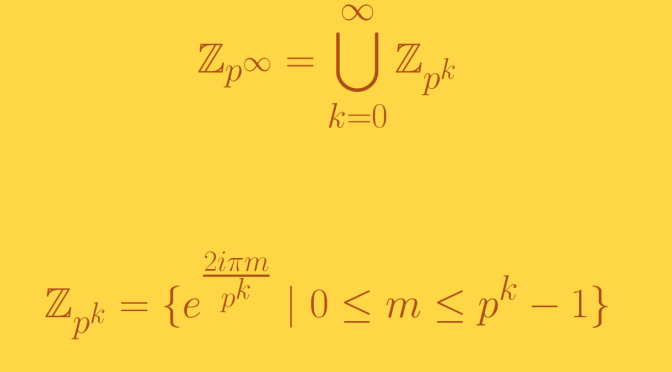# An infinite group whose proper subgroups are all finite

We study some properties of the Prüfer $$p$$-group $$\mathbb{Z}_{p^\infty}$$ for a prime number $$p$$. The Prüfer $$p$$-group may be identified with the subgroup of the circle group, consisting of all $$p^n$$-th roots of unity as $$n$$ ranges over all non-negative integers:
$\mathbb{Z}_{p^\infty}=\bigcup_{k=0}^\infty \mathbb{Z}_{p^k} \text{ where } \mathbb{Z}_{p^k}= \{e^{\frac{2 i \pi m}{p^k}} \ | \ 0 \le m \le p^k-1\}$

### $$\mathbb{Z}_{p^\infty}$$ is a group

First, let’s notice that for $$0 \le m \le n$$ integers we have $$\mathbb{Z}_{p^m} \subseteq \mathbb{Z}_{p^n}$$ as $$p^m | p^n$$. Also for $$m \ge 0$$ $$\mathbb{Z}_{p^m}$$ is a subgroup of the circle group. We also notice that all elements of $$\mathbb{Z}_{p^\infty}$$ have finite orders which are powers of $$p$$.

Now for $$z_1,z_2$$ elements of $$\mathbb{Z}_{p^\infty}$$, there exist $$k_1,k_2 \ge 0$$ with $$z_1 \in \mathbb{Z}_{p^{k_1}}$$ and $$z_2 \in \mathbb{Z}_{p^{k_2}}$$. We can suppose without loss of generality that $$k_1 \le k_2$$. Hence $$z_1, z_2\ \in \mathbb{Z}_{p^{k_2}}$$ and $$z_1 z_2^{-1} \in \mathbb{Z}_{p^{k_2}} \subseteq \mathbb{Z}_{p^\infty}$$ which proves that $$\mathbb{Z}_{p^\infty}$$ is a subgroup of the unit circle.

### All subgroups of $$\mathbb{Z}_{p^\infty}$$ are finite

Let $$H$$ be a proper subgroup of $$\mathbb{Z}_{p^\infty}$$. We prove that $$H$$ is equal to one of the $$\mathbb{Z}_{p^n}$$ for $$n \ge 0$$. If the set of the orders of elements of $$H$$ is infinite, then for all element $$z \in \mathbb{Z}_{p^\infty}$$ of order $$p^k$$, there would exist an element $$z^\prime \in H$$ of order $$p^{k^\prime} > p^k$$. Hence $$H$$ would contain $$\mathbb{Z}_{p^\prime}$$ and $$z \in H$$. Finally $$\mathbb{Z}_{p^\infty}$$ would be included in $$H$$ in contradiction with the hypothesis that $$H$$ is a proper subgroup.

Therefore the set of the orders of the elements of $$H$$ is finite. If $$h \in H$$ is an element with maximum order $$p^n$$, we have $$H = \mathbb{Z}_{p^n}$$.

In conclusion:

• $$\mathbb{Z}_{p^\infty}$$ is an infinite group whose proper subgroups are all finite.
• It is a non-cyclic group whose all proper subgroups are cyclic.